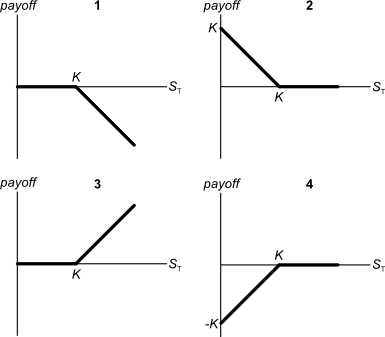# Fight Finance

#### CoursesTagsRandomAllRecentScores

The required return of a project is 10%, given as an effective annual rate. Assume that the cash flows shown in the table are paid all at once at the given point in time.

What is the Net Present Value (NPV) of the project?

 Project Cash Flows Time (yrs) Cash flow ($) 0 -100 1 0 2 121 Below are 4 option graphs. Note that the y-axis is payoff at maturity (T). What options do they depict? List them in the order that they are numberedYou're advising your superstar client 40-cent who is weighing up buying a private jet or a luxury yacht. 40-cent is just as happy with either, but he wants to go with the more cost-effective option. These are the cash flows of the two options: • The private jet can be bought for$6m now, which will cost $12,000 per month in fuel, piloting and airport costs, payable at the end of each month. The jet will last for 12 years. • Or the luxury yacht can be bought for$4m now, which will cost $20,000 per month in fuel, crew and berthing costs, payable at the end of each month. The yacht will last for 20 years. What's unusual about 40-cent is that he is so famous that he will actually be able to sell his jet or yacht for the same price as it was bought since the next generation of superstar musicians will buy it from him as a status symbol. Bank interest rates are 10% pa, given as an effective annual rate. You can assume that 40-cent will live for another 60 years and that when the jet or yacht's life is at an end, he will buy a new one with the same details as above. Would you advise 40-cent to buy the or the ? Note that the effective monthly rate is $r_\text{eff monthly}=(1+0.1)^{1/12}-1=0.00797414$ The coupon rate of a fixed annual-coupon bond is constant (always the same). What can you say about the income return ($r_\text{income}$) of a fixed annual coupon bond? Remember that: $$r_\text{total} = r_\text{income} + r_\text{capital}$$ $$r_\text{total, 0 to 1} = \frac{c_1}{p_0} + \frac{p_1-p_0}{p_0}$$ Assume that there is no change in the bond's total annual yield to maturity from when it is issued to when it matures. Select the most correct statement. From its date of issue until maturity, the income return of a fixed annual coupon: A stock has a beta of 0.5. Its next dividend is expected to be$3, paid one year from now. Dividends are expected to be paid annually and grow by 2% pa forever. Treasury bonds yield 5% pa and the market portfolio's expected return is 10% pa. All returns are effective annual rates.

What is the price of the stock now?

Which of the following statements about the weighted average cost of capital (WACC) is NOT correct?

A fast-growing firm is suitable for valuation using a multi-stage growth model.

It's nominal unlevered cash flow from assets ($CFFA_U$) at the end of this year (t=1) is expected to be $1 million. After that it is expected to grow at a rate of: • 12% pa for the next two years (from t=1 to 3), • 5% over the fourth year (from t=3 to 4), and • -1% forever after that (from t=4 onwards). Note that this is a negative one percent growth rate. Assume that: • The nominal WACC after tax is 9.5% pa and is not expected to change. • The nominal WACC before tax is 10% pa and is not expected to change. • The firm has a target debt-to-equity ratio that it plans to maintain. • The inflation rate is 3% pa. • All rates are given as nominal effective annual rates. What is the levered value of this fast growing firm's assets? Your poor friend asks to borrow some money from you. He would like$1,000 now (t=0) and every year for the next 5 years, so there will be 6 payments of $1,000 from t=0 to t=5 inclusive. In return he will pay you$10,000 in seven years from now (t=7).

What is the net present value (NPV) of lending to your friend?

Assume that your friend will definitely pay you back so the loan is risk-free, and that the yield on risk-free government debt is 10% pa, given as an effective annual rate.

A stock has a beta of 1.5. The market's expected total return is 10% pa and the risk free rate is 5% pa, both given as effective annual rates.

Over the last year, bad economic news was released showing a higher chance of recession. Over this time the share market fell by 1%. The risk free rate was unchanged.

What do you think was the stock's historical return over the last year, given as an effective annual rate?

A one year European-style call option has a strike price of $4. The option's underlying stock pays no dividends and currently trades at$5. The risk-free interest rate is 10% pa continuously compounded. Use a single step binomial tree to calculate the option price, assuming that the price could rise to $8 $(u = 1.6)$ or fall to$3.125 $(d = 1/1.6)$ in one year. The call option price now is: